Форум по искусству и инвестициям в искусствоARTIST-ГРАФИК SILVIA CORDEDDA
 Имя Запомнить? Пароль
 English | РусскийРегистрация Дневники Справка Пользователи Поиск Сообщения за день Все разделы прочитаны

Оценить эту запись

# ARTIST-ГРАФИК SILVIA CORDEDDA

Запись от Про искусство размещена 04.04.2019 в 10:29

FRACTAL GRAPHICS-КОМПЬЮТЕРНОЕ ART

Sylvia Cordedda was born in 1991 in Carrara (Italy). Over a sufficiently short period of time, Sylvia was able to create a number of stunning works of fractal graphics.

Fractal graphics @- view computer graphics. The mathematical basis of fractal graphics @fractal - geometry. The method of imaging should be based on the principle of inheritance from the so-called" родителей" the geometric properties of objects-наследников. Fractal graphics is calculated. The image is based on the equation or system of equations. Therefore, in the memory of the computer to perform all computations, nothing but formulas to keep is not required.

a Basic element of fractal graphics is an equilateral triangle, which was named" фрактальный". In middle part of the sides are constructed equilateral triangles with sides equal to (1/3A) from the source of the fractal triangle. In turn, the average lengths of all the resulting triangles are-наследниками first generation, lining up triangles-наследники second generation of side (1/9a) from the side of the original triangle.

Thus, the smaller elements of the fractal object is repeated the properties of the entire object. The resulting object is called" фрактальной figures". The process of succession can continue indefinitely. Modifying the equation coefficients can be entirely different from each other image, and combining and changing the color of the fractal shapes, it is possible to simulate the images of animate and inanimate nature.

the concept of fractal, fractal geometry and fractal graphics appeared in the late 70-х. Word" фрактал" is derived from the Latin fractus and means" состоящий from fragments". It was proposed by the mathematician Benoit Mandelbrot in 1975 to refer to irregular but self-similar structure, which he did.

Silvia Cordedda for the first time tried themselves in fractal art in the beginning of 2012 and created many fascinating works.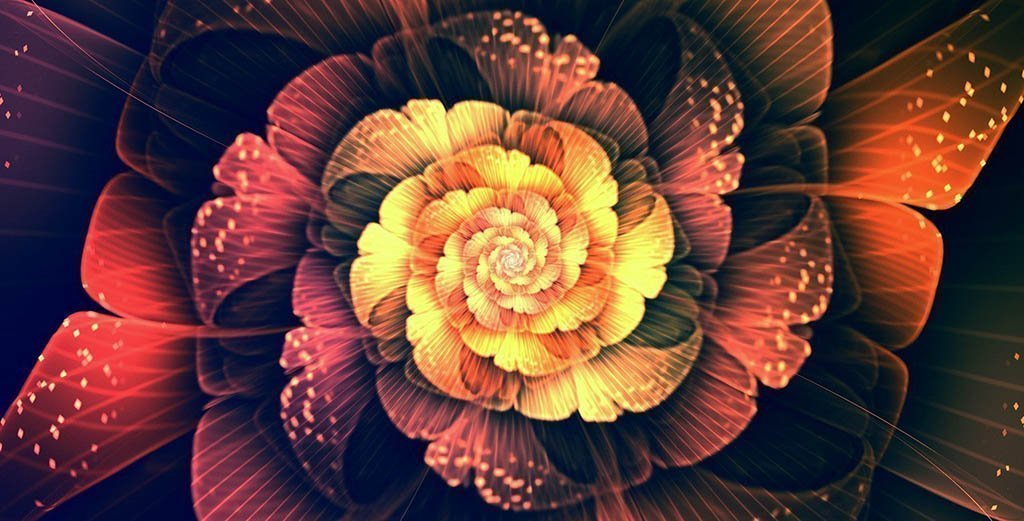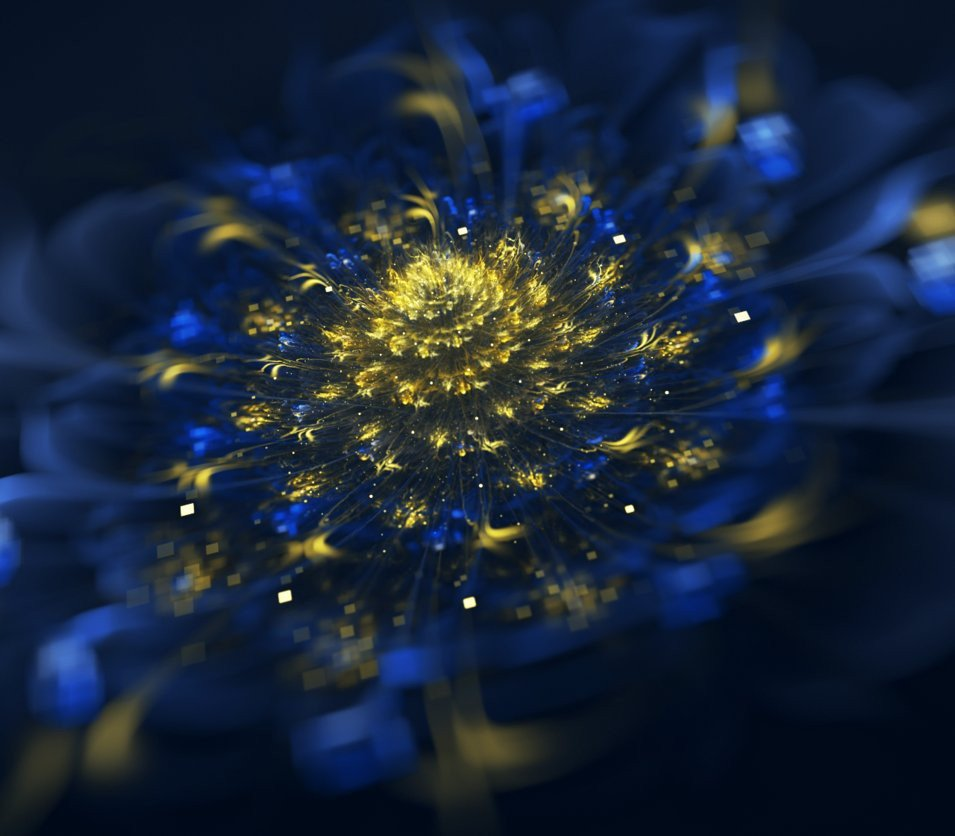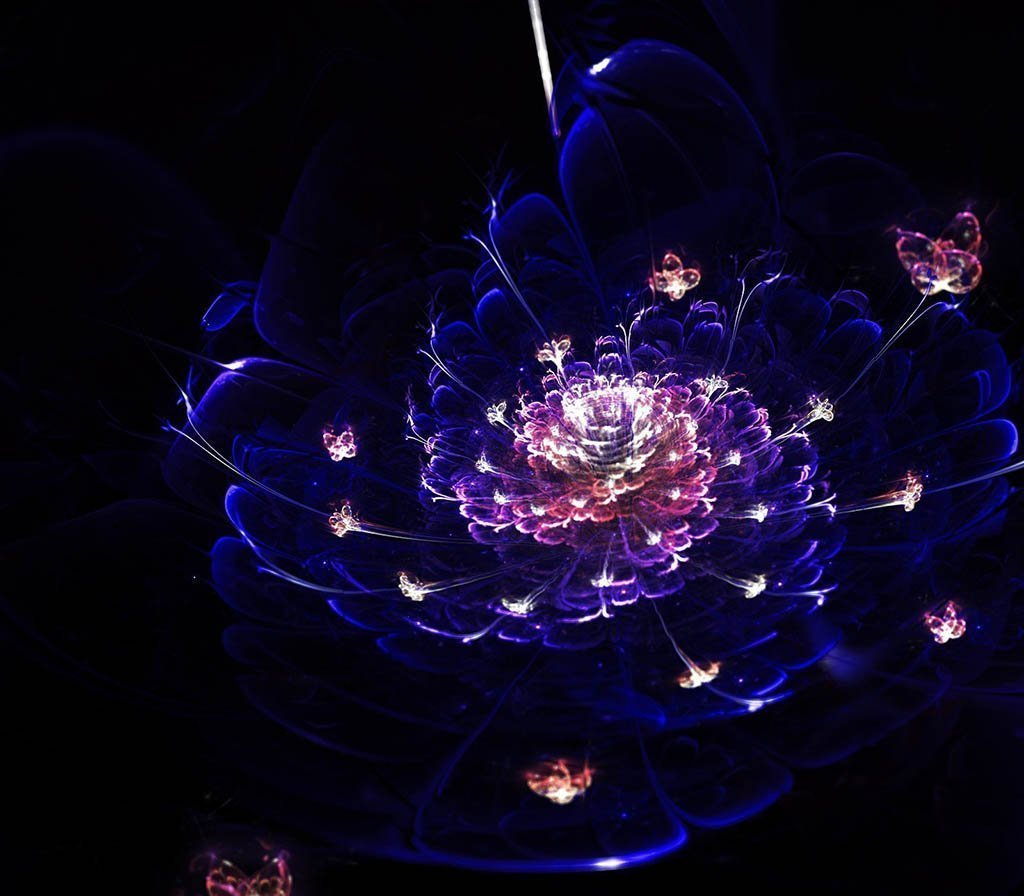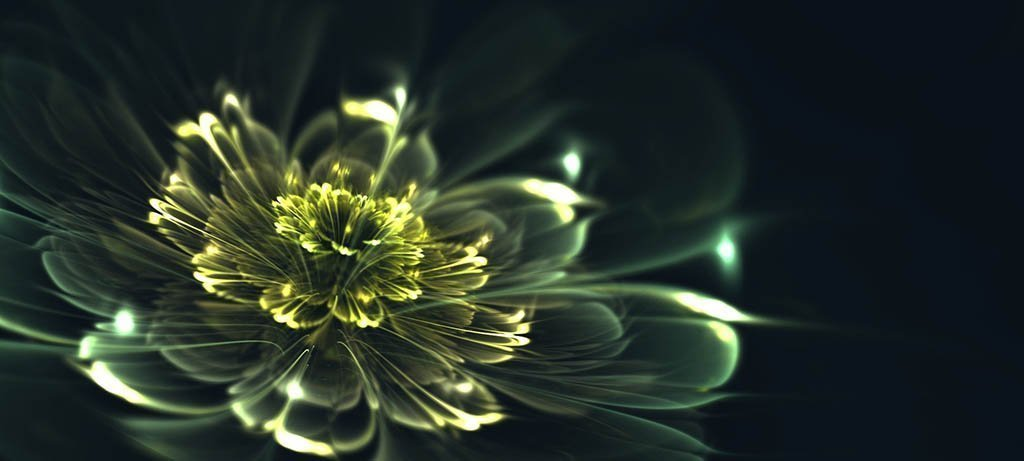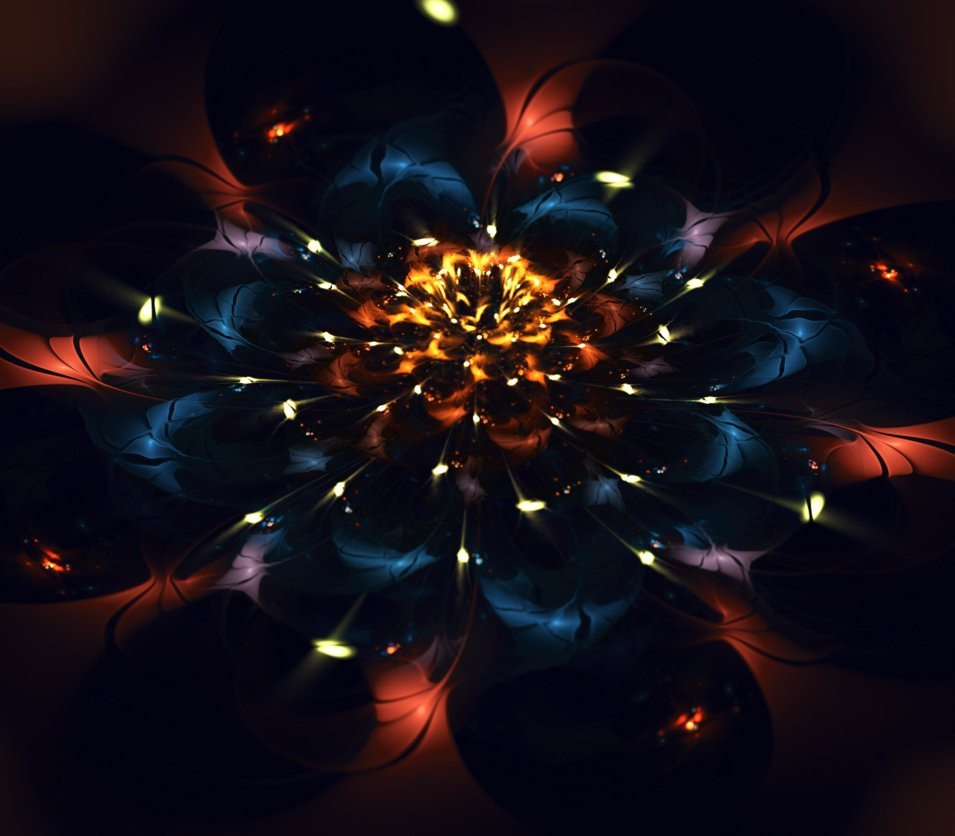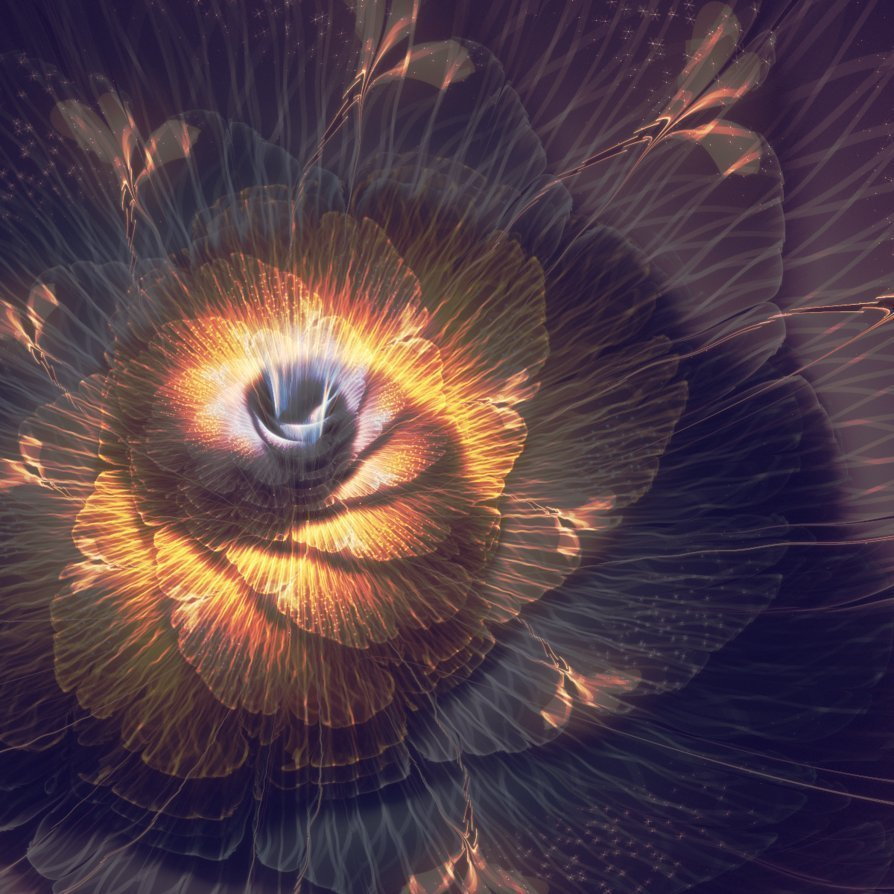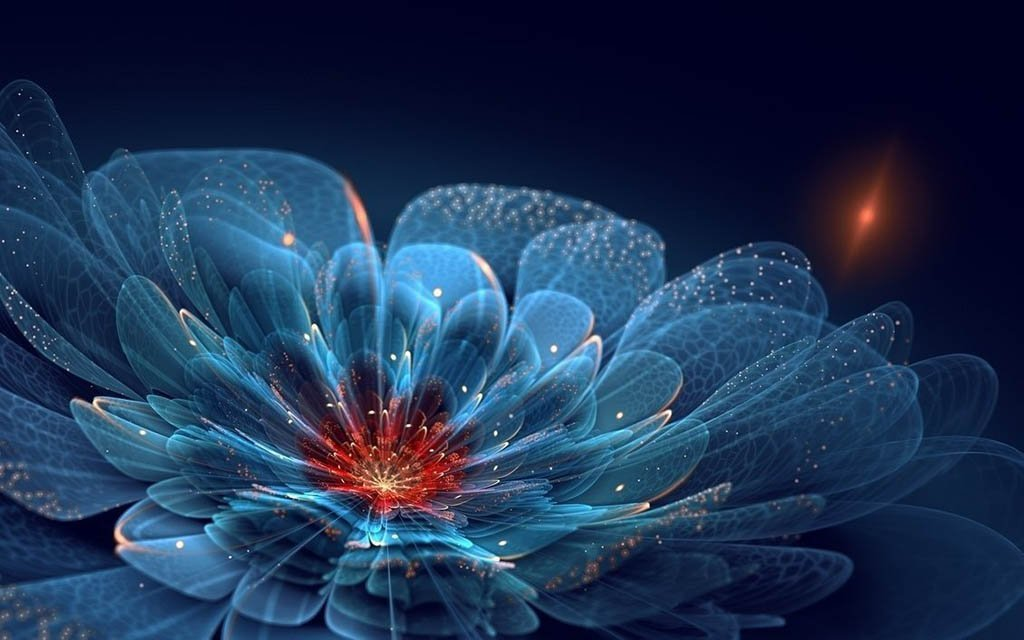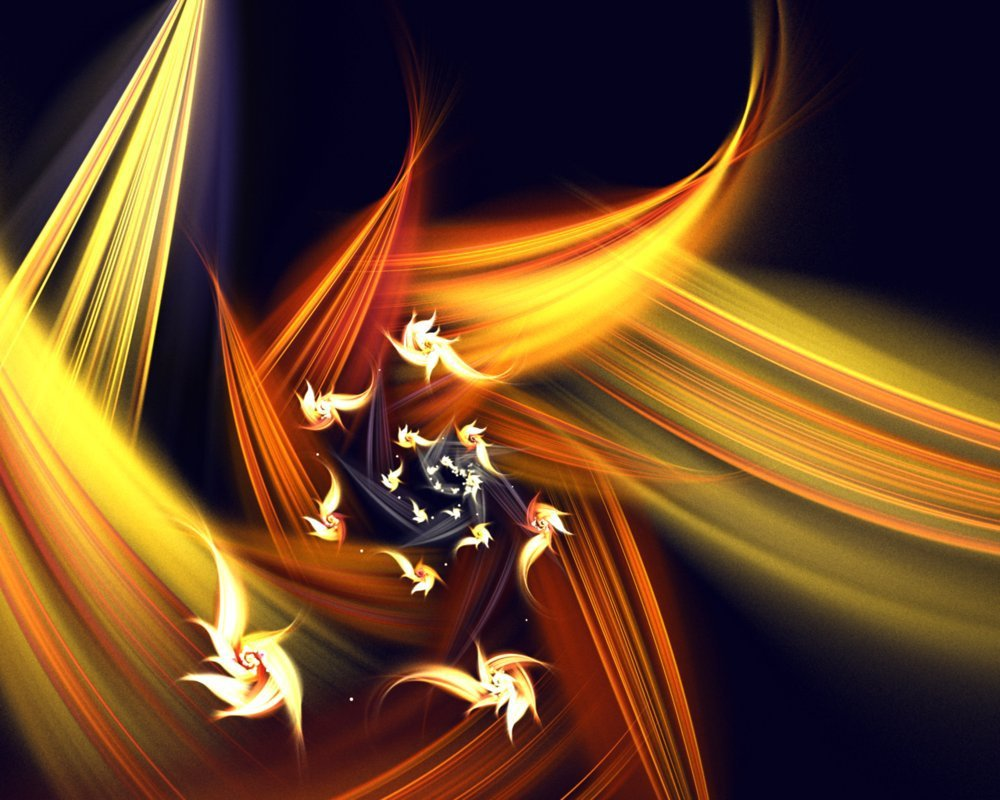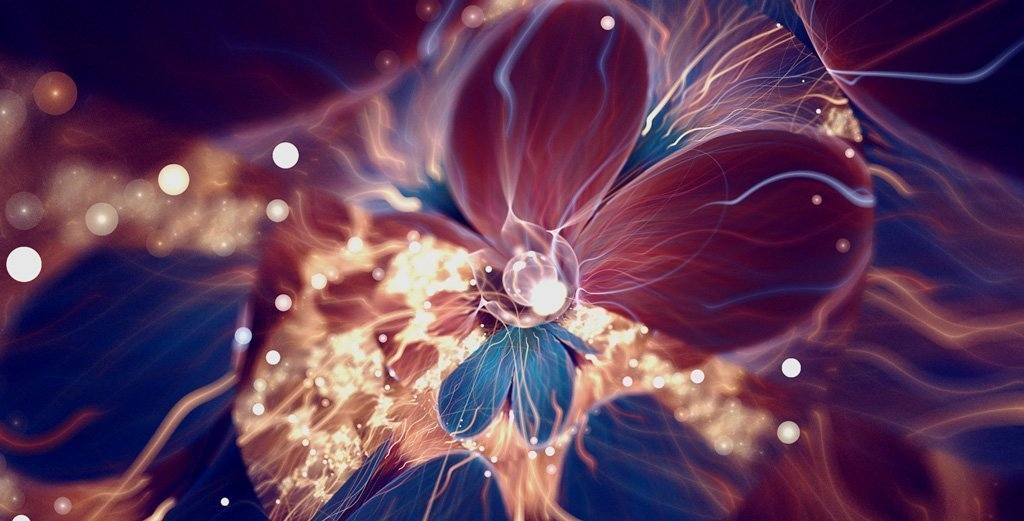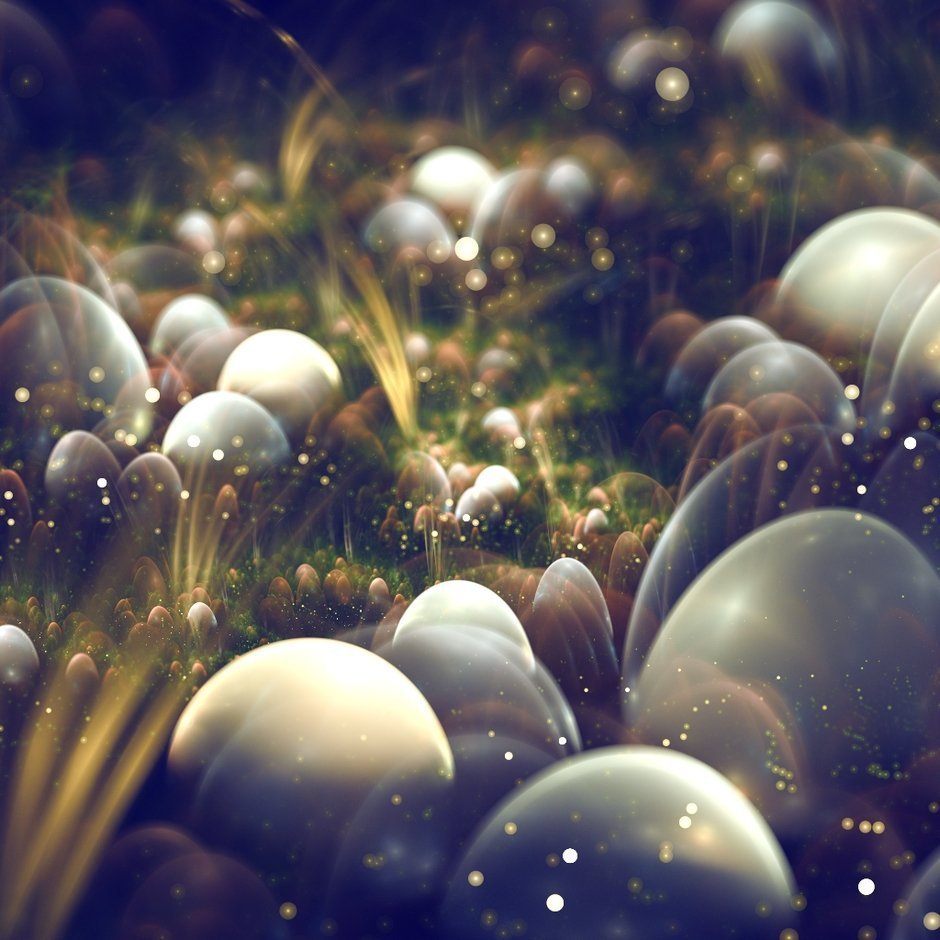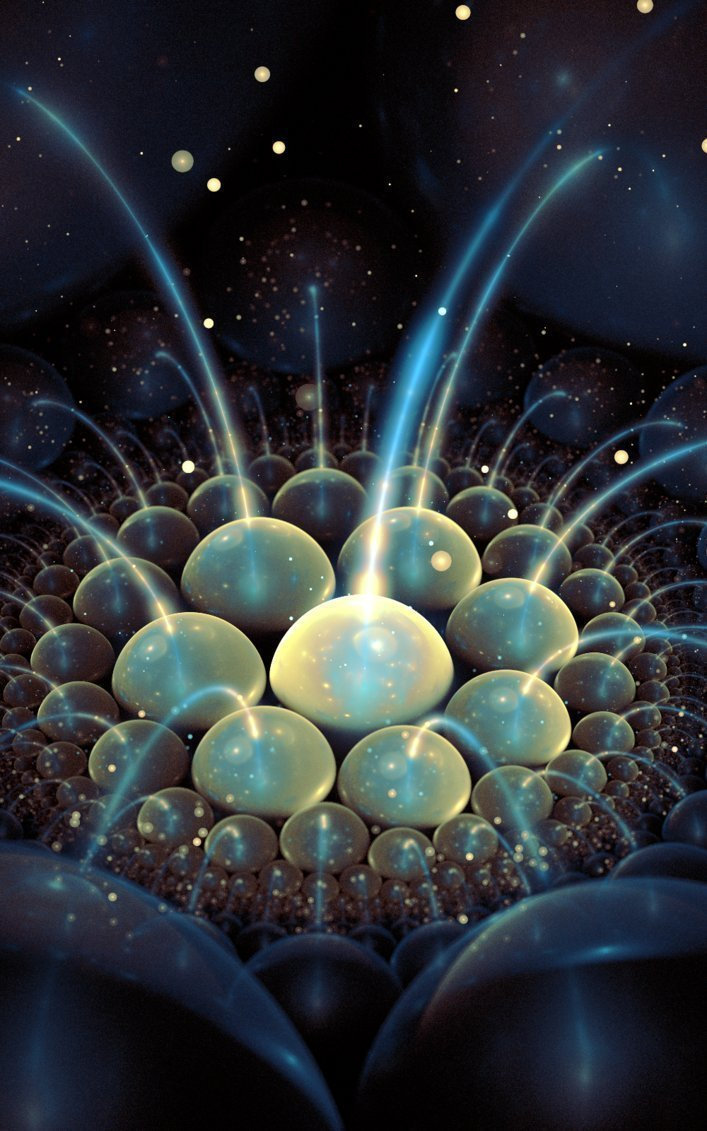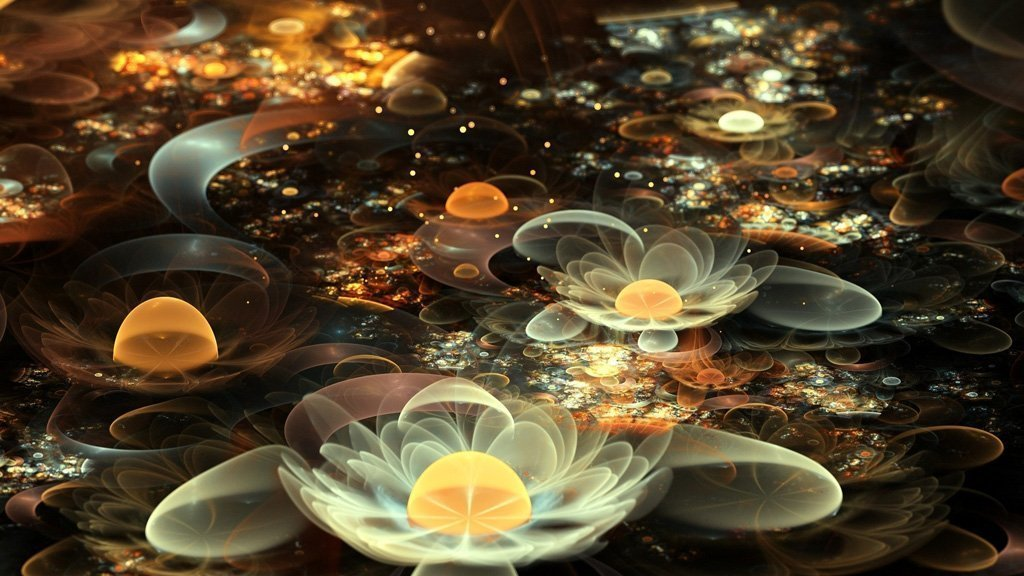http://curious-world.ru/index.php/art/khudozhniki/...a-cordedda-fraktalnaya-grafika

Размещено в Без категории
Просмотров 272 Комментарии 0
Всего комментариев 0

## Комментарии

Часовой пояс GMT +3, время: 16:35.
 -- English (US) -- Russian (RU) Telegram - Instagram - Facebook - Обратная связь - Обработка персональных данных - Архив - Вверх